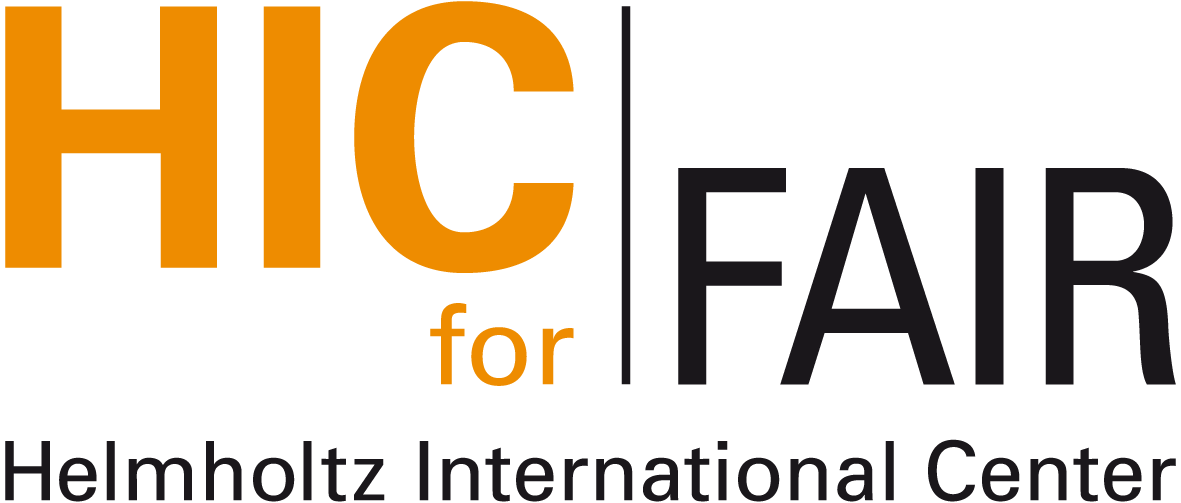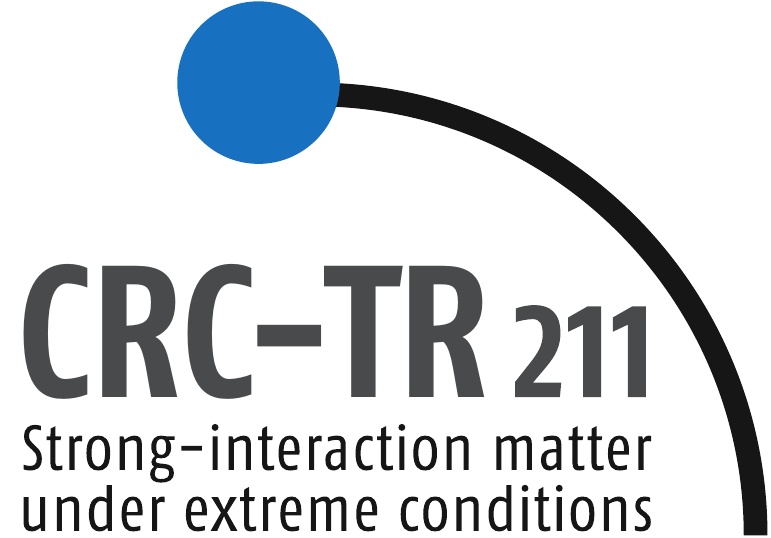#Nuclear Physics Colloquium

Venue: Physics Building, Max-von-Laue-Str. 1, PHYS 02.116
Time: Thursday, November 28, 4:30pm (s.t.)
Contact: hees@itp.uni-frankfurt.de

# Triviality bounds of the O(4) $\boldsymbol{\phi}^4$ model as a theory of the sigma-pion system: lattice vs. continuum

## Gergely Marko (Eötvös University Budapest)

We investigate the apparent contradiction that the O(4) $\phi^4$ model, with parameters set to reproduce the light-meson masses in the vacuum, seem to give practically cutoff independent results in continuum studies, while a simple estimate based on a similar calculation for the Higgs sector by Lüscher and Weisz sets the Landau pole around $\sim 500 \, \text{MeV}$. We carry out a Monte Carlo simulation to determine the triviality bound of the lattice theory precisely and compare results with continuum approximations.

Nuclear Physics Colloquium Homepage